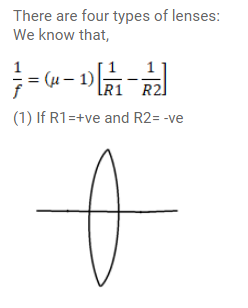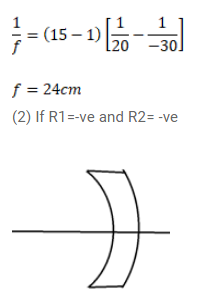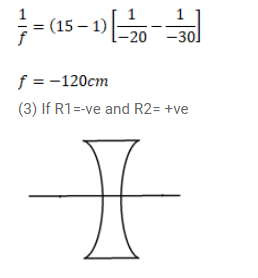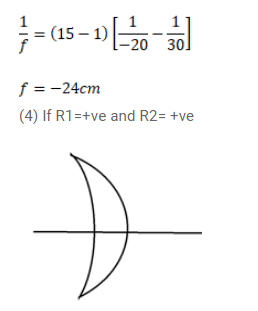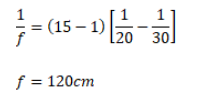# Lenses are constructed by a material of refractive index`
Question:

Lenses are constructed by a material of refractive index $1.50$. The magnitude of the radii of curvature are $20 \mathrm{~cm}$ and $30 \mathrm{~cm}$. Find the focal length of the possible lenses with the above specification.

Solution: